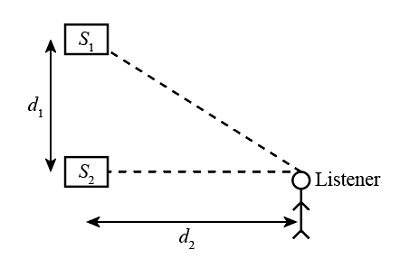# Two loudspeakers, separated by a distance of d1 = 2.34 m, are in phase. Assume the amplitude of...

## Question:

Two loudspeakers, separated by a distance of d{eq}_1 {/eq} = 2.34 m, are in phase. Assume the amplitude of the sound from the speakers is approximately the same at the position of a listener, who is d{eq}_2 {/eq} = 3.84 m directly in front of one of the speakers. Consider the audible range for normal hearing, 20 Hz to 20 kHz.

a. What is the lowest frequency that gives the minimum signal (destructive interference) at the listener's ear?

b. What is the lowest frequency that gives the maximum signal (constructive interference) at the listener's ear? (Take the speed of the sound to be 343 m/s.)

## Interference:

When two waves propagate and superpose with each other, the effect generated by this superposition is termed as interference. Due to this frequency of resultant waves will increase or decrease according to the type of interference.

Given data

• Distance between the two loudspeakers, {eq}{d_1} = 2.34\;{\rm{m}} {/eq}
• Distance between listener and the loudspeaker, {eq}{d_2} = 3.84\;{\rm{m}} {/eq}

Take speed of sound, {eq}c = 343\;{\rm{m/s}} {/eq}(a) Minimum destructive interference occur at {eq}d = \frac{\lambda }{2} {/eq}

Difference in the distance to the listener is,

{eq}\begin{align*} d &= \sqrt {{{3.84}^2} + {{2.34}^2}} - 3.84\\ d &= 0.656\;{\rm{m}} \end{align*} {/eq}

Now lowest frequency that gives minimum signal can be calculated as

{eq}f = \dfrac{c}{\lambda } {/eq}

Wavelength can be calculated as

{eq}\begin{align*} \lambda &= 2d\\ \lambda &= 2 \times 0.656\\ \lambda &= 1.312\;{\rm{m}} \end{align*} {/eq}

Put the value of {eq}\lambda {/eq} in the expression of the frequency, we get

{eq}\begin{align*} f &= \frac{c}{\lambda }\\ f &= \frac{{343}}{{1.312}}\\ f &= 261.43\;{\rm{Hz}} \end{align*} {/eq}

Thus, the lowest frequency that gives minimum signal is {eq}261.43\;{\rm{Hz}}. {/eq}

(b) Constructive interference occur at {eq}d = \lambda = 0.656\;{\rm{m}} {/eq}, so

Maximum frequency can be calculated as

{eq}\begin{align*} f &= \frac{c}{\lambda }\\ f &= \frac{{343}}{{0.72}}\\ f &= 476.38\;{\rm{Hz}} \end{align*} {/eq}

Thus, maximum frequency is {eq}476.38\;{\rm{Hz}} {/eq} .